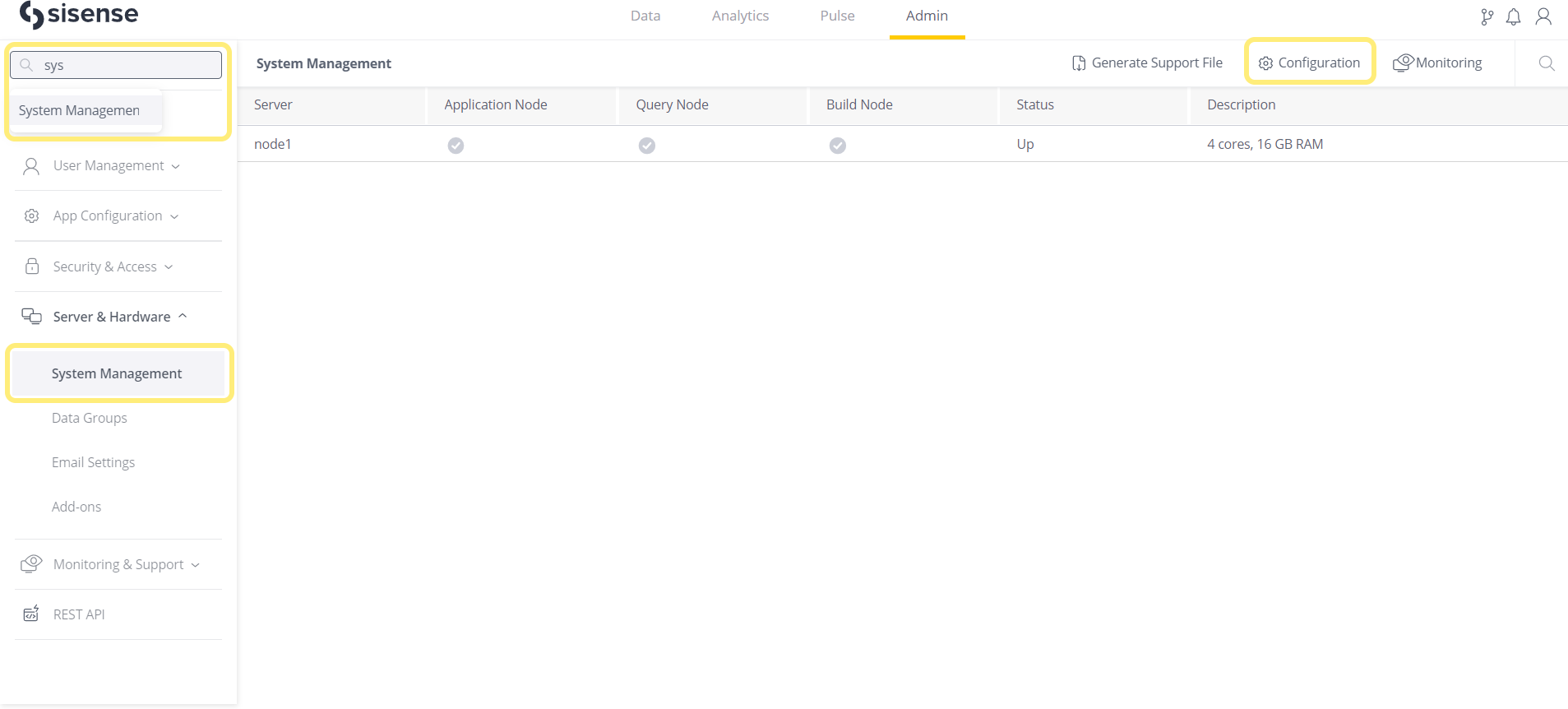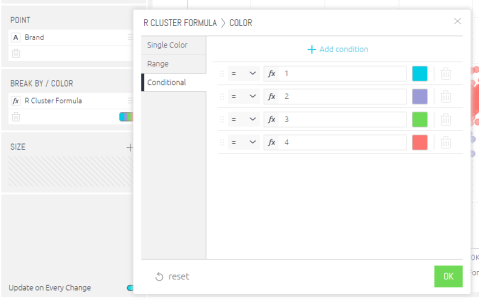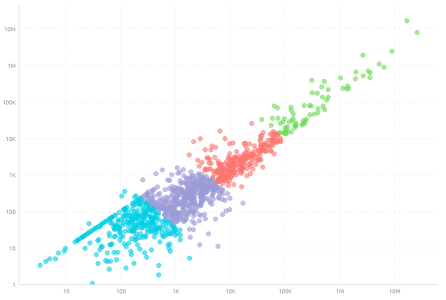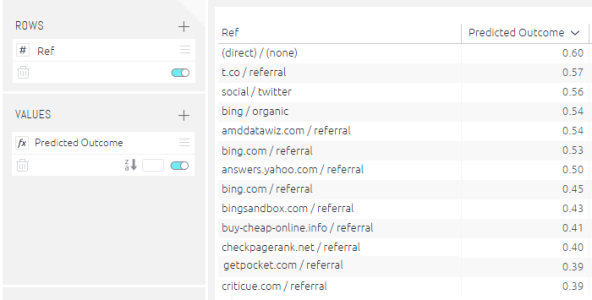# Using R in Formulas

R is a software environment for statistical computing and graphics. Sisense supports the integration of R functions in your formulas.

You can write R code directly in the formula editor, and send fields as parameters. You can also combine R functionality with the regular functions to create advanced and tailored formulas. (To get started with the formula editor and functions, see Creating and Editing a Widget Formula.)

Note:

Before you can integrate R into your formulas, an R server must be set up in your organization.

## Understanding How R works in Sisense

Within a widget, when R code is used and assigned fields as parameters:

1. The selected fields are sent fully to R as a list of arrays.
2. The R code is executed on the R server, referencing the fields as needed.
3. The numeric results from R are sent back to the widget for visualization.

Each request from the R server is handled in a self-contained namespace, so R variables and results cannot be reused across requests, unless they are loaded from a saved location.

R calculations are computed in the R server, and not in the ElastiCube. This means that, for larger data sets, results will not return as fast as they do in regular widgets.

## Connecting Sisense to Your R Server in Linux

1. In the Admin page, search for and select System Management, which is located under Server & Hardware.2. Click Configuration to open the Configuration Manager.
3. Open the ElastiCube Query Params section.
4. In Rserve IP, enter the IP address of your R server.
5. In Rserve Port enter the port of your R server.
6. Click Save to connect to your R server.
7. To use the configured R server with a specific ElastiCube, stop and restart the relevant ElastiCube. For instructions about stopping and starting an ElastiCube, see Working with ElastiCubes.

## Using R functions in Formulas

R code is used in Sisense via two dedicated formulas - RINT & RDOUBLE. Depending on the result type of your R code, use the appropriate function within the Formula Editor, (see Creating and Editing a Widget Formula).

For either formula, RINT or RDOUBLE, the return type has to be an exact match.Multiple numeric field values can be passed as parameters to the R functions that, within your R code, can be accessed via the 'args' argument (see the K-means example below).

Syntax:

`RINT([recycle (true)], [<Ordering>], <R expression>, [<numeric value 1>, ..., <numeric value n>] ) RDOUBLE([recycle (true)], [<Ordering>], <R expression>, [<numeric value 1>, ..., <numeric value n>] )`

Sisense does not support the use of a final ';' in R statements. If you add a semi-colon at the end of your R statement, the code will fail.

Parameters:

• Recycle true/false (default = true) (optional) - This parameter determines whether the results from R are recycled (cached). When set to true, consequent queries are only recalculated if they or the data have changed. Generally, the ElastiCube manages this behavior for all functions. R code might have non-deterministic components (such as randomality functions or date-specific functions). If the R code has non-deterministic components, the ElastiCube may return different execution results, even though the data-set and function have not changed. So, set this to false if your R code contains randomality or other non-deterministic content.

• Ordering (optional) - This sets the sort order of the numeric data sent to R. The Ordering parameter's argument is an index in your data source, or the ORDERING() function. The ORDERING() function arranges the argument values into ascending or descending order. It also breaks ties by further arguments.

For example:

`ORDERING([Total Sales], -1*[COUNT Salesman], MIN(&lt;Office Name&gt;))`

• R expression - Pass your R code here, wrapped in double quotes. R expects the return type to be an array with the same size as the widget's row count. Nulls will be used to make up for shorter arrays, and longer arrays will be trimmed.

When using the Rint/Rdouble functions, use single quotes to wrap strings within your R code. In this way, there will be no double-quote collision with the quotes wrapping your R code within the Rint/Rdouble function.

• Numeric Value Arguments - Numeric values can be passed as arguments to your R code.

• All arguments are passed to R as a 1-based list, named "args". Each item in the list contains an array that represents the field.

For example:

• args[] will return an array that represents the first field that was used as an argument.
• args[] will return the 3rd data value within the 2nd field that was used as an argument.

## Guidelines for using R code in Sisense

For your R code to work well in Sisense, make sure that:

• The resultset returned from R into Sisense is equal in size to the dataset that is sent to R as parameters.
• The order of the resultset returned from R into Sisense matches the incoming order. No assumptions can be made about the incoming order. Do not assume that the incoming order matches the order you see in the widget.

## Example 1: Simple R Function - Kmeans Clustering

Description: In the example below the Total Cost and Total Revenue fields from Sisense are being used to cluster data via a Kmeans function. The result will cluster the data based on the kmeans settings, in this case 4 clusters.

Sisense Syntax:

`RINT(TRUE, "m<- log(matrix(unlist(args), ncol = 2)); kmeans(m,4)\$cluster" ,[Total Cost],[Total Revenue])`

Result: The R expression will return a result from 1 to 4. The widgets color settings can be configured to color corresponding values based on if they equal 1, 2, 3 or 4.

Tip: You can save (star) the complex formula above, and use it again in additional formulas and charts.

In the chart's BREAK BY/COLOR panel, you can change the color of the clusters as well as define the number of clusters and their break points.## Example 2: R Logistic Regression via Sisense

Description: In the example below website traffic data is used to predict if a conversion is likely to occur based on the source of web traffic. The following fields contained in the ElastiCube are passed to the R logistic model: Average Outcome (1=conversion, 0= no conversion), Average Time on Page, Average Pages (viewed) and Average Bounce Rate. This data is passed to a model to predict the outcome and likelihood the traffic will convert.

[For the sake of illustration both the training and test data set are the same in the example]

Sisense Syntax:

`RDOUBLE(TRUE,"mydata<-data.frame(convert=args[], time=args[], pages=args[],``bounce=args[]);lrmodel <- glm(convert time + pages + bounce, data = mydata, family = 'binomial');prob <-``predict(lrmodel, newdata = mydata, type = 'response')", [Average Outcome], [Average TimeonPage], [AveragePages], [Average BounceRate])`

Result: The R expression will return a result from 0 to 1 showing the likelihood of a conversion occurring. Note a similar process can be applied to create a linear regression.Predicted outcome of website conversion based on logistic regression.

Description: In the example below a saved logistic regression built on previous training data is called and used with new website traffic data to predict if a conversion occurs. The following fields contained in the ElastiCube are passed to the saved R logistic model: Average Outcome (1=conversion, 0= no conversion), Average Time on Page, Average Pages (viewed) and Average Bounce Rate. This data is passed to a model to predict the outcome and likelihood the traffic will convert.

Sisense Syntax:

`RDOUBLE(TRUE,"mydata<-data.frame(convert=args[], time=args[], pages=args[],``bounce=args[]);load('C:\rdata');prob <- predict(lrmodel, newdata = mydata, type = 'response')", [Average``Outcome], [Average TimeonPage], [Average Pages], [Average BounceRate])`

Result: The R expression will return a result from 0 to 1 showing the likelihood of a conversion occurring based on the loaded R model in this case stored in 'rdata'.

## Example 4: Building and Saving a R Model using Data from Sisense

Description: In the example below a logistic regression to predict if a website conversion will occur is built using data from Sisense and then saved as an R model. The following fields contained in the ElastiCube are passed to create the R logistic model: Average Outcome (1=conversion, 0= no conversion), Average Time on Page, Average Pages (viewed) and Average Bounce Rate. This data is saved as an rdata file and can be used on other data sets to predict outcomes.

Sisense Syntax:

`RDOUBLE(TRUE,"mydata<-data.frame(convert=args[], time=args[], pages=args[],``bounce=args[]);lrmodel <- glm(convert time + pages + bounce, data = mydata, family = 'binomial');``save(lrmodel, file = 'C:\rdata') ;1", [Average Outcome], [Average TimeonPage], [Average Pages], [Average BounceRate])`

Result: The R expression will use Sisense data to build and save a logistic model in the specified file in this case 'rdata'.